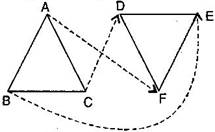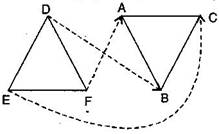### Congruence of Triangles - Solutions

CBSE Class –VII Mathematics

NCERT Solutions
Chapter 7 Congruence of Triangles
(Ex. 7.1)

Question 1. Complete the following statements:

1. Two line segments are congruent if _______________.
2. Among two congruent angles, one has a measure of ${70}^{\circ },$ the measure of other angle is _______________.
3. When we write $\mathrm{\angle }$A = $\mathrm{\angle }$B, we actually mean _______________.

Answer: (a) they have the same length

(b) ${70}^{\circ }$

(c) $m\mathrm{\angle }$A = $m\mathrm{\angle }$B

Question 2. Give any two real time examples for congruent shapes.

(ii) Two teacher’s tables

Question 3. If $\mathrm{\Delta }$ABC $\cong \mathrm{\Delta }$FED under the correspondence ABC $↔$ FED, write all the corresponding congruent parts of the triangles.

Answer: Given: $\mathrm{\Delta }$ABC $\cong$$\mathrm{\Delta }$FED.The corresponding congruent parts of the triangles are:

(i) $\mathrm{\angle }$$↔$$\mathrm{\angle }$F

(ii) $\mathrm{\angle }$$↔$$\mathrm{\angle }$E

(iii) $\mathrm{\angle }$$↔$$\mathrm{\angle }$D

(iv) $\overline{\text{AB}}$$↔$$\overline{\text{FE}}$

(v) $\overline{\text{BC}}$$↔$$\overline{\text{ED}}$

(vi) $\overline{\text{AC}}$$↔$$\overline{\text{FD}}$

Question 4. If $\mathrm{\Delta }$DEF $\cong \mathrm{\Delta }$BCA, write the part(s) of $\mathrm{\Delta }$BCA that correspond to:

i.$\mathrm{\angle }$E

ii.$\overline{\text{EF}}$

iii.$\mathrm{\angle }$F

iv.$\overline{\text{DF}}$

Answer: Given: $\mathrm{\Delta }$DEF $\cong$$\mathrm{\Delta }$BCA.(i) $\mathrm{\angle }$$↔$$\mathrm{\angle }$C

(ii) $\overline{\text{EF}}$$↔$$\overline{\text{CA}}$

(iii) $\mathrm{\angle }$$↔$$\mathrm{\angle }$A

(iv) $\overline{\text{DF}}$$↔$